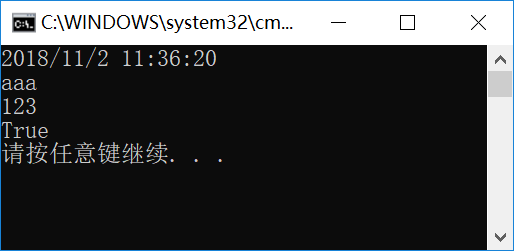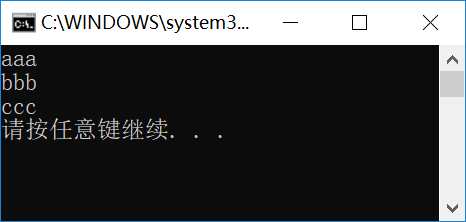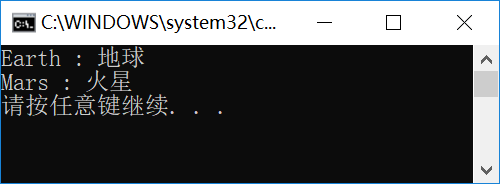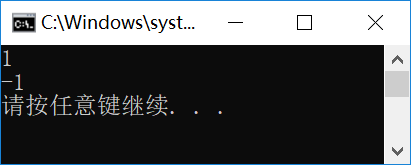• System.Collections命名空间，定义集合处理的非泛型类型。
• System.Collections.Generic命名空间，定义集合处理的泛型类型。

## ArrayList类与List泛型类

ArrayList类和List泛型类用于处理有序的集合，成员使用从0开始的索引值进行访问。下面的代码，我们先来看ArrayList类的基本应用。

``````static void Main(string[] args)
{
ArrayList arr = new ArrayList();
arr.Add("aaa");
arr.Add(123);
arr.Add(true);
arr.Insert(0, DateTime.Now);
//
foreach(object obj in arr)
{
Console.WriteLine(obj.ToString());
}
}
````````````static void Main(string[] args)
{
List<string> lst = new List<string>();
lst.Add("aaa");
lst.Add("bbb");
lst.Add("ccc");
//
for (int i = 0; i < lst.Count; i++)
{
Console.WriteLine(lst[i]);
}
}
``````## Hashtable类和Dictionary泛型类

Hashtable类和Dictionary泛型类，用于处理“键/值”对应的数据类型，即在集合中，每一个键（Key）对应一个值（Value），可以使用键来访问相应的数据值。下面是Hashtable类的基本应用方式。

``````static void Main(string[] args)
{
Hashtable hash = new Hashtable();
hash.Add("Earth", "地球");
hash.Add("Mars", "火星");
Console.WriteLine(hash["Mars"]);
}
``````

Hashtable的键、值都定义为Object类型，这样也就带来了最大的灵活性，和ArrayList的问题一样，当使用不同类型的数据时，可能需要类型的转换工作；而Dictionary泛型类则可以绑定键和值的类型，如下面的代码。

``````static void Main(string[] args)
{
Dictionary<string, string> dict = new Dictionary<string, string>();
dict.Add("Earth", "地球");
dict.Add("Mars", "火星");
Console.WriteLine(dict["Mars"]);
}
``````

``````    static void Main(string[] args)
{
Dictionary<string, string> dict = new Dictionary<string, string>();
dict.Add("Earth", "地球");
dict.Add("Mars", "火星");
//
foreach(string k in dict.Keys)
{
Console.WriteLine("{0} : {1}", k, dict[k]);
}
}
``````## 综合应用

``````using System.Collections.Generic;

namespace ConsoleTest
{
//
public class CDataItem
{
//
public CDataItem(string sName, object oValue)
{
Name = sName;
Value = oValue;
}
//
public string Name { get; set; }
public object Value { get; set; }
}
//
public class CDataList : List<CDataItem>
{

}
}
``````

``````public class CDataList : List<CDataItem>
{
public int Find(string sName)
{
for(int i=0;i<Count;i++)
{
if (string.Compare(sName, this[i].Name, false) == 0)
return i;
}
return -1;
}
}
``````

``````static void Main(string[] args)
{
CDataList lst = new CDataList();
lst.Add(new CDataItem("Earth", "地球"));
lst.Add(new CDataItem("Mars", "火星"));
//
Console.WriteLine(lst.Find("Mars"));
Console.WriteLine(lst.Find("Moon"));
}
``````CHY软件小屋原创作品！